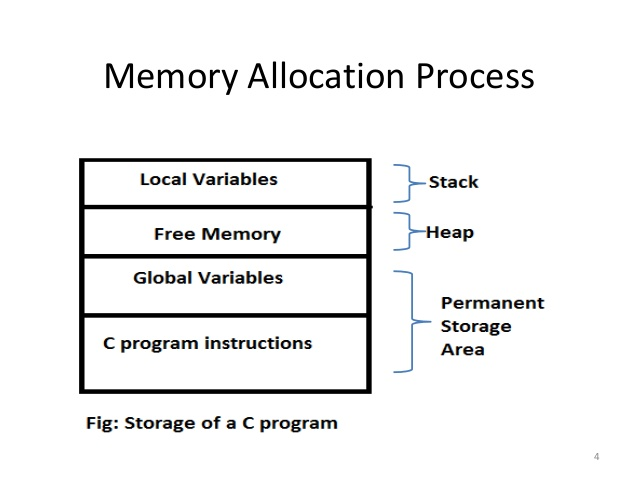×

Search anything:

# Dynamic memory allocation in C

#### C Programming memory allocation dynamic memory Software EngineeringGet this book -> Problems on Array: For Interviews and Competitive Programming

Reading time: 20 minutes | Coding time: 2 minutes

C language requires the number of elements in an array to be specified at compile time. But we may not be able to do so always. Our initial judgement of size, if it is wrong, may cause failure of the program or wastage of memory space.
The process of allocating memory at run time is known as dynamic memory allocation. Although C does not inherently have this facility, there are four librar routines known as "memory managment functions" that can be used for allocating and freeing memory during program execution. They are listed in table below. These functions help us build complex application programs that use the available memory intelligently.

• malloc : Allocates request size of bytes and returns a pointer to the frst byte of the allocated space.
• calloc : Allocates space for an array of elements, initiaizes them to zero and then returns a pointer to the memory.
• free : Frees previously allocated space.
• realloc: Modifies the size of previously allocated space.

### Memory allocation process### Allocating a block of memory: malloc

A block of memory may be allocated using the function malloc. The malloc function reserves a block of memory of specified size and returns a pointer of type void. This means that we can assign it to any type of pointer. It takes the following form:

``````ptr = (cast-type *) malloc(byte-size)
``````

ptr is a pointer of type cast-type.

Example,

`````` x = (int *) malloc (100 *sizeof(int));
``````

On successful execution of this statement, a memory space equivalent to "100 times the size of an int'" bytes is reserved and the address of the first byte of the memory allocated is assigned to the pointer x of type of int.

#### Example

``````#include <stdio.h>
#include <stdlib.h>
int main()
{
int n,i,*p;
printf("Enter number of elements: ");
scanf("%d",&n);
p=(int*)malloc(n * sizeof(int));  //memory allocated using malloc
if(p == NULL)
{
printf("memory cannot be allocated\n");
}
else
{
printf("Enter elements of array:\n");
for(i=0;i<n;++i)
{
scanf("%d",&*(p+i));
}
printf("Elements of array are\n");
for(i=0;i<n;i++)
{
printf("%d\n",*(p+i));
}
}
return 0;
}
``````

### Allocating multiple blocks of memory: calloc

calloc is another memory allocation function that is normally used for requesting memory space at run time for storing derived data types such as arrrays and structures. while malloc allocates a single block of storage space, calloc allocates multiple blocks of storage, each of the same size, and then sets all bytes to zero. The general form of calloc is:

``````ptr = (cast-type *) calloc (n, elem-size);
``````

#### Example

``````#include <stdio.h>
#include <stdlib.h>

int main()
{
int n, i, *ptr, sum = 0;
printf("Enter number of elements: ");
scanf("%d", &n);

ptr = (int*) calloc(n, sizeof(int));
if(ptr == NULL)
{
printf("Error! memory not allocated.");
exit(0);
}

printf("Enter elements: ");
for(i = 0; i < n; ++i)
{
scanf("%d", ptr + i);
sum += *(ptr + i);
}

printf("Sum = %d", sum);
free(ptr);
return 0;
}
``````

### Releasing the used space: free

Dynamically allocated memory created with either calloc() or malloc() doesn't get freed on their own. You must explicitly use free() to release the space.
Syntax:

``````free (ptr);
``````

This statement frees the space allocated in the memory pointed by ptr.

#### Example

``````#include <stdio.h>
#include <stdlib.h>
#include <string.h>

int main(int argc, const char * argv[])
{
char *ptr;
size_t length;
length = 50;

/* Allocate memory for our string */
ptr = (char *)malloc(length);

/* Check to see if we were successful */
if (ptr == NULL)
{
printf("Could not allocate required memory\n");
exit(1);
}
strcpy(ptr, "C malloc at TechOnTheNet.com");
printf("%s\n", ptr);

/* Free the memory we allocated */
free(ptr);

return 0;
}
``````

### Altering the size of a block: realloc

The C library function void realloc(void * ptr, size_t size) attempts to resize the memory block pointed to by ptr that was previously allocated with a call to malloc or calloc.
Syntax:

``````void *realloc(void *ptr, size_t size)
``````

#### Example

``````#include <stdio.h>
#include <stdlib.h>

int main () {
char *str;

/* Initial memory allocation */
str = (char *) malloc(15);
strcpy(str, "burger");
printf("String = %s,  Address = %u\n", str, str);

/* Reallocating memory */
str = (char *) realloc(str, 25);
strcat(str, ".com");
printf("String = %s,  Address = %u\n", str, str);

free(str);

return(0);
}
``````

#### Output

``````String = burger, Address = 355090448
String = burger.com, Address = 355090448
``````

### Advantages of Dynamic memory allocation

• Data structures can grow and shrink according to the requirement.
• We can allocate (create) additional storage whenever we need them.
• We can de-allocate (free/delete) dynamic space whenever we are
done with them.
• Dynamic Allocation is done at run time.

### Disadvantages of Dynamic memory allocation

• As the memory is allocated during runtime, it requires more
time.
• Memory needs to be freed by the user when done. This is important as it is more likely to turn into bugs that are difficult to find.#### Shreya Gupta

Software Development Lead at Developer Student Club Thapar | Intern at OpenGenus | B. Tech in Civil Engineering at Thapar Institute of Engineering and Technology

Dynamic memory allocation in C